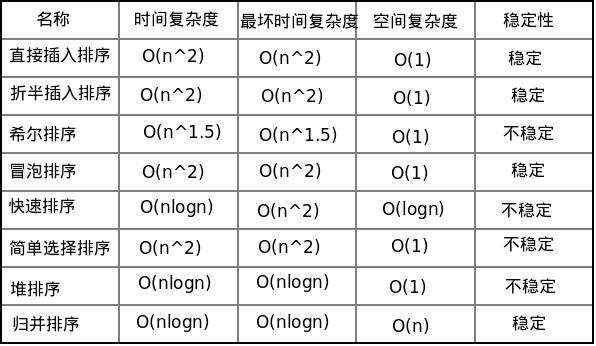# 存档在 2012年3月

## 内部排序算法小结

2012年3月20日

### 5.总结1.直接插入排序、折半插入排序、冒泡排序和简单选择排序合称为简单排序，它们的时间复杂度均为O(n^2)，空间复杂度为O(1)。稳定性方面只有简单选择排序是不稳定的排序算法。简单排序只适合n较小的情况。

2.当待排序记录基本有序时，直接插入排序是最佳的排序算法。它常与快速排序和归并排序等结合使用，因为这些算法会将整个打排序记录划分为若干个子记录，这些子记录数量较小而且基本有序。

3.排序性能较好的有希尔排序、快速排序、堆排序和归并排序，除了希尔排序的时间复杂度为O(n^1.5)，其他排序算法均为O(nlogn)。虽然快速排序最坏情况下时间性能下降为O(n^2)，但是就平均性能而言快速排序算法是最好的。堆排序和归并排序的性能比较稳定，当n比较大时，归并排序的性能比堆排序要好，但是它需要的辅助空间却为O(n)。

4.所有的排序算法中，不稳定的算法有简单选择排序、希尔排序、快速排序和堆排序。性能较好的排序算法中只有归并排序是稳定的。排序是按记录的主关键字进行的,此时不用考虑排序方法的稳定性。如果排序是按记录的次关键字进行的, 则应充分考虑排序方法的稳定性。

## 选择类排序

2012年3月17日

### 1.简单选择排序

```void selectSort(int *r, int length)
{
int i, j, k, n;

n = length;

for ( i = 1; i <= n - 1; i++) {
k = i;

for ( j = i + 1; j <= n; j++)
if (r[j] < r[k])
k = j;
if ( k != i) {
r = r[i];
r[i] = r[k];
r[k] = r;
}
output(r, length);
}
}
```

### 2.堆排序

```void heapSort(int *r, int length)
{
int i, n;
int t;

create_heap(r, length);

n = length;

for ( i = n; i >= 2; i--) {
t = r;
r = r[i];
r[i]= t;
output(r, length);
}
}
```

```void create_heap(int *r, int length)
{
int i, n;

n = length;

for ( i = n / 2; i >= 1; i--)
}```

```void adjust_heap(int *r, int k, int m)
{
int i, j, t;
int finished;

r = r[k];
i = k;
j = 2 * i;

finished = 0;

while (j <= m && !finished) {
if (j < m && r[j] < r[j + 1])
j++;
if (r >= r[j])
finished = 1;
else {
r[i] = r[j];
i = j;
j = 2 * i;
}
}

r[i] = r;
}
```

## 交换类排序

2012年3月16日

### 1.冒泡排序

```void bubbleSort(int *r, int length)
{
int n, change;
int i, j, k;

n = length;
change = 1;

for ( i = 1; i <= n - 1 && change; i++) {
change = 0;
for ( j = 1; j <= n - i; j++)
if (r[j] > r[j + 1]) {
r = r[j];
r[j] = r[j + 1];
r[j + 1] = r;
change = 1;
}
output(r, length);
}
}
```

### 2.快速排序

```void quickSort(int *r, int low, int high, int length)
{
int pos;

if (low < high) {
pos = quickPass(r, low, high);
quickSort(r, low, pos - 1, length);
quickSort(r, pos + 1, high, length);
output(r, length);
}
}
```

quickPass()用于实现一趟快速排序，一趟快速排序结束的条件是low不小于high。可以看到快速排序中是将low和high两个不相邻位置的元素进行交换。

```int quickPass(int *r, int left, int right)
{
int low, high;

low = left;
high = right;
r = r[low];

while (low < high) {
while (low < high && r[high] >= r)
high--;
if (low < high) {
r[low] = r[high];
low++;
}

while (low < high && r[low] <= r)
low++;
if (low < high) {
r[high] = r[low];
high--;
}
}

r[low] = r;
return low;
}
```

## 插入类排序

2012年3月15日

### 1.直接插入排序

```void insSort(int *r, int length)
{
int i, j;

printf("Sorting:\n");
for ( i = 2; i <= length; i++) {
r = r[i];
j = i - 1;

while (r < r[j]) {
r[j + 1] = r[j];
j--;
}

r[j + 1] = r;

output(r, length);
}
}
```

### 2.折半插入排序

```void binSort(int *r, int length)
{
int i, j;
int low ,high, mid;

printf("Sorting:\n");
for ( i = 2; i <= length; i++) {
r = r[i];
low = 1;
high = i - 1;

while (low <= high) {
mid = (low + high) / 2;
if (r < r[mid])
high = mid - 1;
else
low = mid + 1;
}

for (j = i - 1; j >= low; j--)
r[j + 1] = r[j];
r[high + 1] = r;
output(r, length);

}
}
```

### 3.希尔排序

```void shellSort(int *r, int length, int *delta, int n)
{
int i;
for ( i = 0; i < n; i++) {
shellInsert(r, length, delta[i]);
}
}
```

```void shellInsert(int *r, int length, int d)
{
int i, j;
int k;

for (i = 1 + d; i <= length; i++) {
if (r[i] < r[i - d]) {
r = r[i];

for (j = i - d; j > 0 && r < r[j]; j -= d) {
r[j + d] = r[j];
}
r[j + d] = r;
}
}
output(r, length);
}```

## 伙伴算法的实现-释放页框

2012年3月12日

```#define __free_page(page) __free_pages((page), 0)

void __free_pages(struct page *page, unsigned int order)
{
if (put_page_testzero(page)) {
if (order == 0)
free_hot_cold_page(page, 0);
else
__free_pages_ok(page, order);
}
}```

### 1.__free_pages_ok()

```static void __free_pages_ok(struct page *page, unsigned int order)
{
…………
free_one_page(page_zone(page), page, order, get_pageblock_migratetype(page));
…………
}

static void free_one_page(struct zone *zone, struct page *page, int order,int migratetype)
{
spin_lock(&zone->lock);
zone->all_unreclaimable = 0;
zone->pages_scanned = 0;

__mod_zone_page_state(zone, NR_FREE_PAGES, 1 << order);
__free_one_page(page, zone, order, migratetype);
spin_unlock(&zone->lock);
}```

### 2.__free_one_page()

```static inline void __free_one_page(struct page *page,
struct zone *zone, unsigned int order,
int migratetype)
{
unsigned long page_idx;

if (unlikely(PageCompound(page)))
if (unlikely(destroy_compound_page(page, order)))
return;

VM_BUG_ON(migratetype == -1);

page_idx = page_to_pfn(page) & ((1 << MAX_ORDER) - 1);

VM_BUG_ON(page_idx & ((1 << order) - 1));

while (order < MAX_ORDER-1) {
unsigned long combined_idx;
struct page *buddy;

buddy = __page_find_buddy(page, page_idx, order);
if (!page_is_buddy(page, buddy, order))
break;

list_del(&buddy->lru);
zone->free_area[order].nr_free--;
rmv_page_order(buddy);
combined_idx = __find_combined_index(page_idx, order);
page = page + (combined_idx - page_idx);
page_idx = combined_idx;
order++;
}
set_page_order(page, order);
&zone->free_area[order].free_list[migratetype]);
zone->free_area[order].nr_free++;
}
```

### 3.__page_find_buddy()

```static inline struct page *
__page_find_buddy(struct page *page, unsigned long page_idx, unsigned int order)
{
unsigned long buddy_idx = page_idx ^ (1 << order);

return page + (buddy_idx - page_idx);
}```

### 4.__find_combined_index()

```static inline unsigned long
__find_combined_index(unsigned long page_idx, unsigned int order)
{
return (page_idx & ~(1 << order));
}```